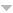## Publications

Export 60 results:
Sort by: Author Title Type [ Year]
2021
Coelho, Carlos A., Filipe J. Marques, Nadab Jorge, and Célia Nunes. "On the distribution of the likelihood ratio test of independence for random sample size - a computational approach." Journal of Computational and Applied Mathematics (2021): 113394. AbstractWebsite

The test of independence of two groups of variables is addressed in the case where the sample size N is considered randomly distributed. This assumption may lead to a more realist testing procedure since in many situations the sample size is not known in advance. Three sample schemes are considered where N may have a Poisson, Binomial or Hypergeometric distribution. For the case of two groups with p1 and p2 variables, it is shown that when either p1 or p2 (or both) are even the exact distribution corresponds to a finite or an infinite mixture of Exponentiated Generalized Integer Gamma distributions. In these cases a computational module is made available for the cumulative distribution function of the test statistic. When both p1 and p2 are odd, the exact distribution of the test statistic may be represented as a finite or an infinite mixture of products of independent Beta random variables whose density and cumulative distribution functions do not have a manageable closed form. Therefore, a computational approach for the evaluation of the cumulative distribution function is provided based on a numerical inversion formula originally developed for Laplace transforms. When the exact distribution is represented through infinite mixtures, an upper bound for the error of truncation of the cumulative distribution function is provided. Numerical studies are developed in order to analyze the precision of the results and the accuracy of the upper bounds proposed. A simulation study is provided in order to assess the power of the test when the sample size N is considered randomly distributed. The results are compared with the ones obtained for the fixed sample size case.

2020
Ferreira, Johannes Theodorus, Andriëtte Bekker, Filipe Marques, and M. Laidlaw. "An Enriched $\alpha$- $μ$ Model as Fading Candidate." Mathematical Problems in Engineering. 2020 (2020). Abstract
n/a
Ghosh, Indranil, Filipe Marques, and Subrata Chakraborty. "A new bivariate Poisson distribution via conditional specification: properties and applications." Journal of Applied Statistics (2020): 1-23. Abstract
n/a
Coolen, Frank PA, and Filipe J. Marques. "Nonparametric Predictive Inference for Test Reproducibility by Sampling Future Data Orderings." Journal of Statistical Theory and Practice. 14 (2020): 1-22. Abstract
n/a
Marques, FJ, and CA Coelho. "Testing simultaneously different covariance block diagonal structures–the multi-sample case." Journal of Applied Statistics (2020): 1-20. Abstract
n/a
2019
Marques, Filipe, Frank Coolen, and Tahani Coolen-Maturi. "Approximations for the likelihood ratio statistic for hypothesis testing between two Beta distributions." Journal of Statistical Theory and Practice. 13 (2019): 17. Abstract
n/a
Marques, Filipe J., Frank PA Coolen, and Tahani Coolen-Maturi. "Introducing nonparametric predictive inference methods for reproducibility of likelihood ratio tests." Journal of Statistical Theory and Practice. 13 (2019): 15. Abstract
n/a
Marques, Filipe J., Theodor M. Loots, and Andriëtte Bekker. "Series representations for densities functions of a family of distributions—Application to sums of independent random variables." Mathematical Methods in the Applied Sciences. 42 (2019): 5718-5735. Abstract
n/a
2018
Marques, Filipe J. "Gamma-Series Representations for the Sum of Independent Gamma Random Variables and for the Product of Independent Beta Random Variables." Recent Studies on Risk Analysis and Statistical Modeling. Springer, Cham, 2018. 241-253. Abstract
n/a